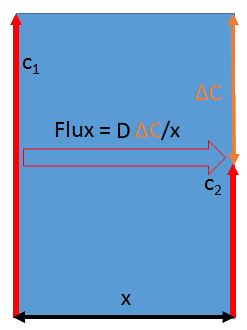## Diffusion Basics

### Quick Start

The basic idea behind diffusion is simple and intuitive. Our problems arise because we end up with a bit of calculus, which many of us don't find easy to interpret.

Here we show that the equations aren't so hard and that a calculus-free equation can already give us some helpful insights from simple diffusion data. Knowing the thickness, L, of a sample, the saturated concentration, c, of a solvent in the polymer (not the solubility of the polymer in solvent!), and some rough idea of a Diffusion Coefficient, D,(we'll see where we can find some values), we can say how much stuff (flux, F) will flow through the polymer, and the time, t½, needed to reach half that flux.

Or, more usefully, if you know L and can plot F as a function of time, by sliding D till you get the correct t½ you've found your diffusion coefficient!

### Basics

L (μm)
C (Vol Fraction)
D (cm²/s)
*10^
F (g/cm²/s)
t½ (s)The formula to calculate diffusion is simplicity itself and is called Fick's First Law. It says that the amount of material per unit area that will flow in a given time (the Flux, F) over a given distance x depends on a constant, the Diffusion Coefficient D and the change of concentration ΔC over that distance (the concentration gradient), i.e. ΔC/x. Therefore:

F=DΔC/x (in calculus notation, F=DδC/δx)

Note that purists add a negative sign on the right-hand side. Fick's Second Law of Diffusion is not quite so intuitive. It tells us that the change of concentration with time is the change of flux with distance. In other words:

ΔC/t=ΔFlux/x = Δ(DΔC/x)/x (in calculus notation δC/δt=Dδ²C/δ²x)

Although these two laws are simple, they are differential equations (for example, the concentration gradient changes with the flux which depends on the concentration gradient) and the solutions in most real cases (where D also depends on concentration) are not straightforward. Therefore numerical simulations are required to capture what really happens. Fortunately on this page we can explore some cases which happen to be very relevant in practical terms. Once we're happy with the basics we can then look at more general cases, with the app doing all the hard work of carrying out the numerical simulations.

### Steady state diffusion across a barrier

We already know the answer to how much chemical will diffuse across a barrier of thickness L if the concentration is C at one side and 0 at the other. We have a concentration gradient (C-0)/L, a diffusion coefficient D, so the flux F is:

F=DC/L

The real problem is not the formula, but getting inputs and outputs in the right units. D is usually expressed in terms of cm²/s and has values of 10-5 for self diffusion in liquids, thru 10-7 for diffusion through rubbery polymers up to 10-12 for typical polymers and 10-15 for very close-packed polymers. If you have a D value in m²/s divide it by 104. Concentrations are best expressed in terms of g/cm³. Thicknesses are typically in µm but have to be converted to cm for the calculation. Fluxes are, therefore, in g/cm²/s. A complication is that it's often easier to think of solubility in terms of volume fraction. Assuming, for simplicity, that all densities are 1g/cc, a volume fraction of 1 equals a concentration of 1g/cc. This is convenient. It is intuitive to think of a polymer containing a 0.1 fraction of a solvent (i.e. 10%) and this simply becomes 0.1g/cc for the calculations. Adjusting for relative densities is a trivial task.

### Half Time

In addition to the Flux, the time, t½, to reach half the equilibrium amount (either by absorption from empty or desorption from equilibrium) is given by t½=0.049L²/D. This equation follows from the fact that absorption or desorption goes as the square root of time. It is often used to calculate the diffusion coefficient from experimental half-time measurements.

Now we can see what happens as we change our key parameters. Note that because of the limitations of app interfaces the diffusion coefficient is entered as a value between 1 and 9.9 and an exponent from 10-5 to 10-15. Because of the plane of symmetry in the middle of a free film, L=Thickness/2 when modeling absorption or desorption where mass is transported through both surfaces. When modeling permeation, which is one-sided exposure, then L=Thickness.